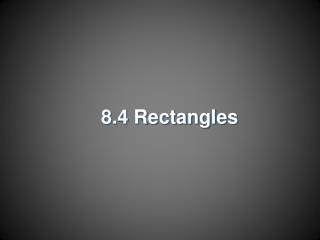DownloadDownload Presentation8.4 Rectangles

# 8.4 Rectangles

Download Presentation## 8.4 Rectangles

- - - - - - - - - - - - - - - - - - - - - - - - - - - E N D - - - - - - - - - - - - - - - - - - - - - - - - - - -
##### Presentation Transcript

1. 8.4 Rectangles

2. Objectives • Recognize and apply properties of rectangles • Determine whether parallelograms are rectangles

3. Rectangles • A rectangle is a parallelogram with four right angles.

4. Rectangles • Since rectangles are parallelograms, they have all their properties: • Opposite sides are || and ≅. • Opposite s are ≅. • Consecutive s are supplementary. • Diagonals bisect each other. • In addition, there exists Theorem 8.13 which states if a is a rectangle then the diagonals are ≅.

5. Quadrilateral RSTU is a rectangle. If and find x. Example 1:

6. The diagonals of a rectangle are congruent, Example 1: Definition of congruent segments Substitution Subtract 6x from each side. Add 4 to each side. Answer: 8

8. Example 2a: Quadrilateral LMNP is a rectangle. Findx.

9. Example 2a: Angle Addition Theorem Substitution Simplify. Subtract 10 from each side. Divide each side by 8. Answer: 10

10. Example 2b: Quadrilateral LMNP is a rectangle. Findy.

11. Example 2b: Since a rectangle is a parallelogram, opposite sides are parallel. So, alternate interior angles are congruent. Alternate Interior Angles Theorem Substitution Simplify. Subtract 2 from each side. Divide each side by 6. Answer: 5

13. Kyle is building a barn for his horse. He measures the diagonals of the door opening to make sure that they bisect each other and they are congruent. How does he know that the corners are angles? We know that A parallelogram with congruent diagonals is a rectangle. Therefore, the corners are angles. Answer: Example 3:

14. Answer: Since opposite sides are parallel, we know thatRSTU is a parallelogram. We know that . A parallelogram with congruent diagonals is a rectangle. Therefore, the corners are Your Turn: Max is building a swimming pool in his backyard. He measures the length and width of the pool so that opposite sides are parallel. He also measures the diagonals of the pool to make sure that they are congruent. How does he know that the measure of each corner is 90?

15. Method 1:Use the Slope Formula, to see ifconsecutive sides are perpendicular. Example 4: Quadrilateral ABCD has vertices A(–2, 1), B(4, 3), C(5, 0), and D(–1, –2). Determine whether ABCD is a rectangle using the Slope Formula.

16. quadrilateral ABCD is a parallelogram. The product of the slopes of consecutive sides is –1. This means that Example 4: Answer: The perpendicular segments create four right angles. Therefore, by definition ABCD is a rectangle.

17. Method 2:Use the Distance Formula, to determine whether opposite sides are congruent. Example 4:

18. Example 4: Since each pair of opposite sides of the quadrilateral have the same measure, they are congruent. Quadrilateral ABCD is a parallelogram.

19. The length of each diagonal is Example 4: Find the length of the diagonals. Answer: Since the diagonals are congruent, ABCD is a rectangle.

20. Your Turn: Quadrilateral WXYZ has vertices W(–2, 1), X(–1, 3), Y(3, 1), and Z(2, –1).Determine whether WXYZ is a rectangle using the Distance Formula.

21. Answer: we can conclude that opposite sides of the quadrilateral are congruent. Therefore, WXYZ is a parallelogram. Diagonals WY and XZ each have a length of 5. Since the diagonals are congruent, WXYZ is a rectangle by Theorem 8.14. Your Turn: